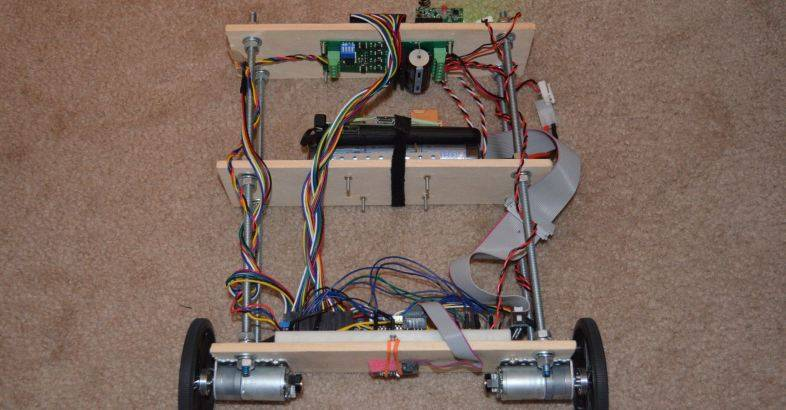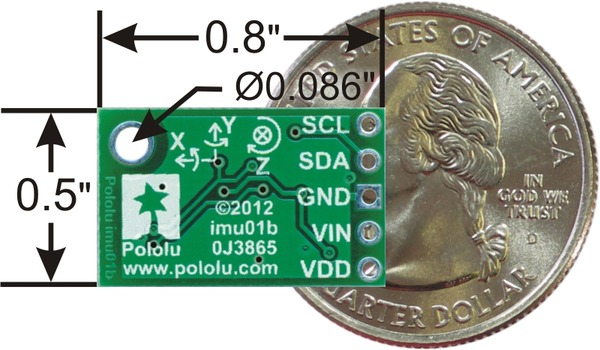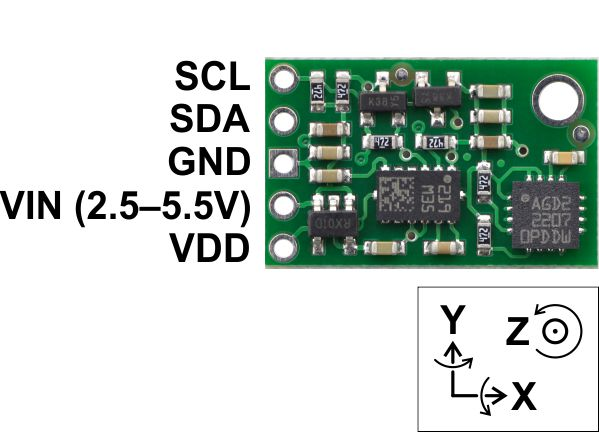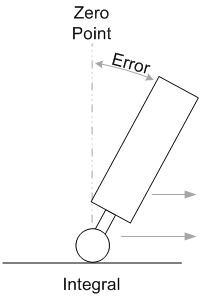# Success with a Balancing Robot using a Raspberry Pi

I have had success with getting PiBBOT to balance.
` `

` `

` `

` `

When I saw my first two wheel balancing robot I was very fascinated.  And after receiving my Raspberry Pi, I decided to build one myself.
PiBBOT (Pi Balancing roBOT) is my first successful balancing robot. And it has room for improvement and extra functionality.
` `
When building PiBBOT, I had a few roadblocks I needed to overcome;

1. I originally had the Anker as the power source for both Pi and the motors, however the amperage was too low.
2. Original H-Bridge not powerful enough for my motors
3. Gyro calculation off by 15 degrees

` `
The TFT displays the angles from the accelerometer, gyro, complementary filter and power to the motors.
The buttons are to turn the motors on and off and to reset the gyro.
` `What next?

• Incorporate the wheel encoders for better balance
• Build a sturdier frame
• Implement direction control
• The able to self right itself after falling over
• Room mapping
• Retrieve a can of beer from the fridge

` `
PiBBOT consists of these components;
TFT; 2.2″ 18-bit color TFT LCD display

Pololu;
Motors;  9.7:1 Metal Gearmotor 25Dx48L mm with 48 CPR Encoder
Wheels; Pololu Wheel 90x10mm
IMU; MinIMU-9 v2 Gyro, Accelerometer, and Compass (L3GD20 and LSM303DLHC).
` `
Anker;
Battery; Anker® Astro3 10000mAh  5V / 9V / 12V 2A Dual USB Output External Battery
` `

Tenergy;
Battery; 7.2V Tenergy 3800mAh Flat NiMH High Power

## Theory

The basics behind a balancing robot is based on the Inverted Pendulum concept. The goal is to have a control algorithm called Proportional Integral Derivative (PID) to keep the robot balanced by trying to keep the wheels under the center of gravity. Eg. If the robot leans forwards, the wheels spin forward trying correct the lean.

One axis of an accelerometer and one axis from a gyroscope are used to measure the current angle and the rate of rotation. A well timed looped is needed to keep track of everything.
Calculations are then done to provide power via PWM to the motors and in the right direction to keep the robot upright.
` `
` `

## Angle Measurement

To measure the angles, I use the MinIMU-9 v2 Gyro, Accelerometer, and Compass inertial measurement unit(IMU) from Pololu.This is a digital IMU and can be read using I2C. It is also powered off the Raspberry Pi 3v pin.

Here you can see the 3 individual I2C addresses;

pi@raspberrypi ~ \$ sudo /usr/sbin/i2cdetect -y 1
0 1 2 3 4 5 6 7 8 9 a b c d e f
00: — — — — — — — — — — — — —
10: — — — — — — — — — 19 — — — — 1e —
20: — — — — — — — — — — — — — — — —
30: — — — — — — — — — — — — — — — —
40: — — — — — — — — — — — — — — — —
50: — — — — — — — — — — — — — — — —
60: — — — — — — — — — — — 6b — — — —
70: — — — — — — — —
pi@raspberrypi ~ \$

` `
When using the IMU to calculate the angle, readings from both the gyro and accelerometer are needed, which are then combined. This is because using either on their own will result in inaccurate readings.
` `
Here is why;
Gyro – A gyro measures the rate of rotation, which has to be tracked over time to calculate the current angle. This tracking <and noise> causes the gyro to drift.  However, gyros are good at measuring quick sharp movements.
` `
Accelerometer – Accelerometers are used to sense both static (e.g. gravity) and dynamic (e.g. sudden starts/stops) acceleration. They don’t need to be tracked like a gyro and can  measure the current angle at any given time. Accelerometers however are very noisy and are only useful for tracking angles over a long period of time.
` `
` `
The gyro and accelerometer have a number of sensitivity levels.  For the gyro I am using a measurement range of 250dps, which has a sensitivity of is 0.00875 dps/LSB. And the accelerometer I am using 8 g full scale, 4 mg/LSB and FS = 10.

Later I will discuss how to combine the angles from both sensors.
` `
` `
` `

## Converting the RAW gyro and accelerometer values to something useful.

The gyro I use is a L3GD20

For the gyro, we need to work out how fast it is rotating in degrees per second(dps). We then need to track the angle deviation from point zero. Point zero is when the PiBBOT is upright and balanced. We then need to track this over time.
` `
Gyro Rotation Rate;
` `
rate_gyr_x = (float) gyr_x_raw * G_GAIN
` `
` `

gyr_x_raw = raw data acquired from the gyro X axis.
G_GAIN  = 0.00875, which is based off the sensitivity level used by the gyro.
rate_gyr_x =  the rate of rotation per second.
` `

` `
Gyro Angle Tracking
` `
gyroXangle+=rate_gyr_x * DT
` `
DT = loop period.
gyroXangle = is the current X angle calculated from the gyro X data.

I have DT set top 0.02, which is 20ms. This is how long it takes to complete one cycle of the main loop. This loop period has to be constant and accurate, otherwise your gyro will drift.
` `
` `

Accelerometer Angle
` `
The accelerometer I use is LSM303DLHC
The X angle can be calculated by using trigonometry and the raw values from the accelerometer Y and Z axis. This is done using the Atan2 function to return the principal value of the tangent of Y and Z, expressed in radians.
` `

We add π to the radians so that we get a result between 0 and 2. We then convert the radians to degrees by multiplying the radians by 57.29578 (180/π).
` `
` `
M_PI = 3.14159265358979323846
` `
` `

## Combining the angles

Once you have the both X angles, you will have to combined them to overcome the gyro drift and the accelerometer noise.
We can do this by using a filter, which will trust the gyro for short periods of time and the accelerometer for longer periods of time.
` `
There are two filers you could use, the Kalman Filter or the Complementary Filter.   I used the Complementary filter as it is simple to understand and less CPU intensive.   The Kalman filter is way to far complex and would be very processor intensive.

` `
Complementary filter;
` `
Current angle = 98% x (current angle + gyro rotation rate) + (2% * Accelerometer angle)

or

CFangleX=AA*(CFangleX+rate_gyr_x*DT) +(1 – AA) * AccXangle;
` `
` `

AA = 0.98  Complementary filter constant

CFangleX is our final angle which will be used to balance PiBBOT.
` `
` `
In summary, our main piece of code for angles is;

```rate_gyr_x = (float) gyr_x_raw * G_GAIN;
CFangleX=AA*(CFangleX+rate_gyr_x*DT) +(1 - AA) * AccXangle;```

` `
` `

## Proportional Integral Derivative (PID)

A PID control algorithm is used to balance the robot by driving the motors based on the tilt.The proportional(P) term of the PID is based on the current angle difference or error from Point Zero (where robot balances) multiplied by the P gain. The P gain is a number we need to tweak to get the right amount of proportional control.
The proportional control will try and correct the balance based on the current error from point zero.
` `
` `The portion of code for the P term is;
` `
Pterm = KP * CFangleX;
` `
The integral (I) term of the PID is based on the current angle difference or error from Point Zero multiplied by the I gain, which is that accumulated over time. The integral control assist in balancing the robot if it is moving.The portion of code for the I term is;
` `
iTerm += KI * CFangleX;
` `
The derivative (D) term of the PID is based on the current rate of rotation. It is used to dampen the response as the robot reaches Zero Point. Without the derivative control, the robot will overshoot and than start to oscillate.The portion of code for the D term is;
` `
dTerm = KD *  (CFangleX – lastAngle);
lastAngle = CFangleX;

` `
` `
The addition of all the PID values will be used to drive the motors in the right direction and with the right power;
` `
output = Pterm + iTerm + dTerm;
` `
Getting the PID values correct(KP,KI,KD) is the hardest part of building PiBBOT.
` `
The PID values need to be manually tuned;

1. Set KI and KD to zero.
2. Set KP high enougth so that the motors drive the wheels under the robot in the direction it is falling. The robot should overshoot Point Zero a little bit and then start to oscillate.
3. Reduce KP by about 10% to so you get just below the oscillation.
4. Increase KI. This will help the robot reach Point Zero faster and will also start to oscillate. Try and get a value so that the robot just oscillates
5. Increase KD to dampen the oscillation and until the robot balances.

` `
It is best to change these values while trying to balance to see the results in real time, a potentiometer could be used for this. I have used the RF module to increase or decrease the values.
` `
` `
` `
` `

## 36 thoughts on “Success with a Balancing Robot using a Raspberry Pi”

1. Grant Johnston says:

This is great, its a really good use of a very small Pi. The BBC have an article about a Segway style platform with an Ipad on the top that you can remotely control. This could be a DIY version.

Are you going to package up the code….please.

1. cheers.
Regarding the code, I will post it.. maybe under another entry on how to read the values from the IMU.
I still need to clean it up a bit.

2. Rob says:

You have followed a very similar path to my own project earwig robot . I agree the Kalman is overkill and the complementary does just as good a job in less time . My mix was about 98/2 also.
So encoders next. …you will have a lot of backlash in the gearbox so the encoder can show the velocity spike . you could filter in SW but I prefer to use belt drive with maxon coreless DC motors . They are the DB’s, world class stall torque which is off the blocks acceleration, and if you can gain >1g acceleration you can lift to the verticle. if your wheels grip, carpet will hold over 1 coifficient of friction so >1g forward accel can be achived, iso standards all =1. ,. I think mapping is the great horizon, the raspberry pi chip i like because its got loads of memory ontop of the CPU, you can develope on it . the error can never end on angle it should have velocity=0 as a goal.

I have seen earwig, and it balances very well.. and very precise.
I think a may start to look at new motors. What model number are your Maxon motors?

3. Jay K says:

Hello, this is a great project.
Luckily I am also working on interfacing the Raspberry Pi with the same gyro that you have used.I am also using an accelermeter from Sparkfun(https://www.sparkfun.com/products/10955). To combine the accelerometer and the gyroscope values, I am using the same Complementary filter. The problem where I am stuck is that time DT is coming out to be quite high. So all my calculations are generating undesired results. This is my code (https://github.com/smithakamat/Hipi_Accelerometer)….It would be great if you could share your code, so I could know where am I going wrong. I have to submit this project in school in a few days, and I am stuck in the gyro angle part.

4. Jayz says:

Hi this is great explanation. Can you post your code about angle calculation from IMU.
Thanks

5. Christian Sievers says:

Hallo, i see a problem in your calculations for the closed loop. There is no sample time in your equations. For example it should be iTerm += KI * CFangleX * sampletime. But to be hornest, I don’t know how to realize a constant sampling on the pi. May be there is the possibility of using realtime linux.

1. The sample time is not really needed in the PID equation.
Sample time is only needed to get the gyro rate of rotation, you can see this in;
gyroXangle+=rate_gyr_x * DT.

I somewhat disagree with your comment regarding sample time. A real-time Unix OS would differently be better; however I am able to get my code to run at a constant 20ms loop. It actually runs at 6-7ms, but I add a delay to get to 20ms. The gyro drift is only about 2 degrees every 15 secs, this is very, very, very good from a \$35 computer. And the complementary filter cancels this drift anyway.
If the gyro was drifting 2 degrees every 3 seconds, then I would look for another platform.
In the video, there is some instability, however this is from motor backlash.

6. Larry Cuba says:

I don’t see a mention of the structural system. What’s that ErectorSet-like system that you’re using?

1. Larry Cuba says:

terrific. thanks.

7. ARM says:

hi,

how to use that code for arduino users?.

thank you

8. Johnny says:

check this out http://www.engineers-excel.com/Apps/PID_Simulator/Equations.htm
(You can see engineers actually use kI= 1/I rather than kI=I it might help your tuning that way round, easier 10 or 100 rather than 0.1 or 0.001)

that ref suggests integral term something like [excuse my syntax]

Iterm=Ki*(Iold + CfAngleX*tstep)
Iold=Iold + CfAngleX*tstep

or I guess equivalently

Iold=Iold+CfAngleX*tstep
Iterm=Ki*Iold

…where tstep is the time step.
When tstep is constant, like you had, it can be ignored and then effectively becomes absorbed into KI

…and I think is a little different from your formula
iTerm += KI * CFangleX;
My version only applies KI to the ‘new’ integral, yours saves it as part of the old integral.
I’m not quite sure whether that will make much difference in reality, but it will affect the numerical value of KI you end up with.

9. What is the chassis made of? I mean those plates with many holes for screws, and all these things. Couldn’t find them in shops.

10. And do the holes and spacings between them fit all the things you connected with screws? I mean the pololu motor brackets and screw holes on the PCBs.

1. I had no issues with the motor brackets, but I had to improvise when connecting the PCB

11. HoaNT says:

Great work!
I dont know if PID is right if we put some object to one side of the balancing bot. Do we need to tune PID values again?
Thank you so much.

12. Can you explain why you took he full 2000 dps scale on the gyro ? I assume 1000 dps would be more than enough but would come with the advantage of a
a higher resolution.

Nice one. May I get the circuit diagram for all connections? I’m still new in using Raspberry Pi

14. zack says:

the output of the PID will attach to the speed of the motor right? do you get the output value in range of 0-255?

1. the output I use wasd -128 to +128. Anything in the negative was reverse.

15. trungvn says:

hi, can you give me program PID for self robot?? Thank you
!

16. nicola says:

Hi , good work , but……

your floor is very helpfull …… if yuo try on table the results will not the same…..

Nicola.

17. foo says:

Awesome!
Thanks for explanation and especially the code!

18. Lenny says:

What motor driver are you using and how do you control your motor? SoftPWM, HardwarePWM or with I2C (saw your github code). Thanks!

19. Harry says:

Hello Guys,

I have decided to build this robot to learn electronics/programming.

got a couple of questions. Is this possible to make this robot using the sense hat?
What did you use to build the frame of the robot?

Thanks heaps

20. Hi!
This post has been really helpful! Very well explained.
Im building from scratch a raspberry pi based quadcopter and now I want to code my PID controller.
My doubt is about how to control the loop times, frequency,…
How did you do in your project?
Thanks!!

This site uses Akismet to reduce spam. Learn how your comment data is processed.